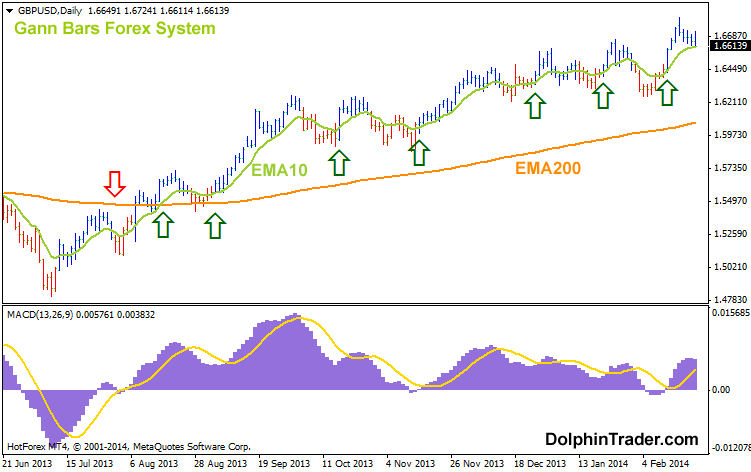#### Tag Archive

Tag Archives for " exponential moving average "

## 3 Exponential (3 EMAs) Moving Average Metatrader 4 Indicator

This is a very nice buy/sell forex indicator based on the crossover of 3 exponential moving averages. A green signal to buy appears when the short-term EMA crosses the medium-term EMA and long-term EMA from below and the medium-term EMA lies above the long-term EMA. A red signal to sell appears when the short-term EMA […]

## 2 EMA – 7 EMA Guppy Multi Moving Average Metatrader 4 Indicator

This is the eleventh GMMA forex indicator in a series of 11 indicators entirely based on the Guppy multi moving average. The RainbowMMA_10 consists of the following moving averages: 7 period exponential moving average, 6 period exponential moving average, 5 period exponential moving average, 4 period exponential moving average, 3 period exponential moving average and […]

## 8 EMA – 13 EMA Guppy Multi Moving Average Metatrader 4 Indicator

This is the tenth GMMA forex indicator in a series of 11 indicators entirely based on the Guppy multi moving average. The RainbowMMA_10 consists of the following moving averages: 13 period exponential moving average, 12 period exponential moving average, 11 period exponential moving average, 10 period exponential moving average, 9 period exponential moving average and […]

## 14 EMA – 23 EMA Guppy Multi Moving Average Metatrader 4 Indicator

This is the ninth GMMA forex indicator in a series of 11 indicators entirely based on the Guppy multi moving average. The RainbowMMA_09 consists of the following moving averages: 23 period exponential moving average, 21 period exponential moving average, 19 period exponential moving average, 17 period exponential moving average, 15 period exponential moving average and […]

## 37 EMA – 50 EMA Guppy Multi Moving Average Metatrader 4 Indicator

This is the seventh GMMA forex indicator in a series of 11 indicators entirely based on the Guppy multi moving average. The RainbowMMA_07 consists of the following moving averages: 50 period exponential moving average, 47 period exponential moving average, 44 period exponential moving average, 41 period exponential moving average, 39 period exponential moving average and […]

## 175 EMA – 200 EMA Guppy Multi Moving Average Metatrader 4 Indicator

The Guppy Multi Moving Average Indicator (GMMA), developed by Daryl Guppy, is a trend following indicator made up of a set of moving averages. This is the first indicator in a series of 11 indicators entirely based on the Guppy multi moving average. The RainbowMMA_01 consists of the following moving averages: 200 period exponential moving […]

3

## 200 Pips Daily Forex Chart Strategy With 3 EMA’sTrading off the daily chart with 3 exponential moving averages system and forex buy/sell oscillator. Our aim is to make 200 pips on each trade. This simple system requires very little maintenance. You’ll only need to check your charts once a day. Chart Setup Indicators: 25 EMA, 60 EMA, 100 EMA, Robby DSS Forex Preferred […]

## Profitable Forex Scalping System With 2 EMA’s And Trading Oscillator

Here’s another profitable forex scalping system with 2 EMA cross and the Schaff Trend Cycle oscillator. We aim for 15 pips per trade. Simply no greed. Just 15 pips. Chart Setup Indicators: 40 exponential moving average, 110 exponential moving average, schaff-trend-cycle Preferred time frame(s): M1 Trading sessions: Euro, US Preferred Currency pairs: GBP/USD, GBP/JPY Download […]

## Gann Bars Forex SystemA trend following system based on the cross-over of 2 exponentional moving averages with MACD and the Gann indicator. This system enables traders to execute multiple trades in the direction of an established market trend. Chart Setup Indicators: Gann HiLo activator bars, 200EMA, 10EMA, MACD (13,26,9) Preferred time frame(s): M30, H1, H4, Daily, Weekly Trading sessions: […]